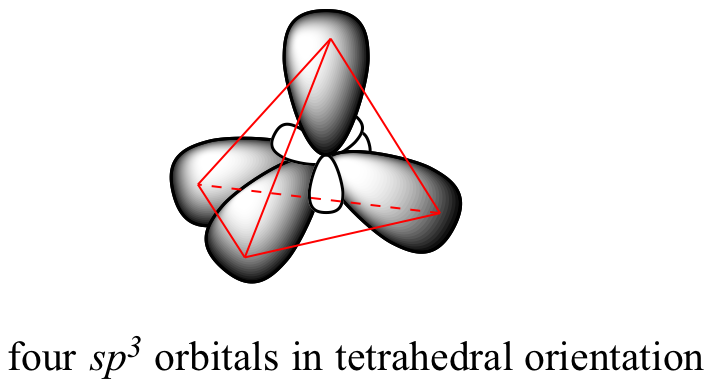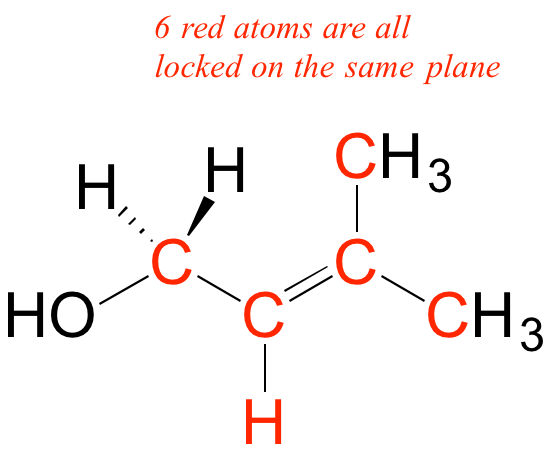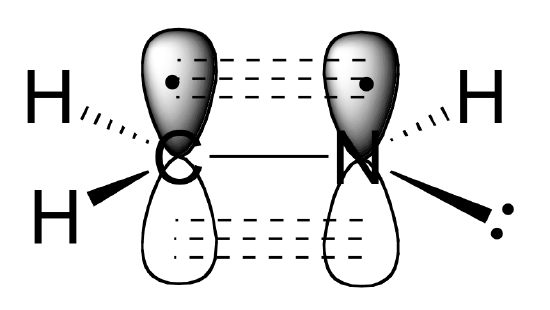# 2.2: Hybrid orbitals

$$\newcommand{\vecs}{\overset { \rightharpoonup} {\mathbf{#1}} }$$ $$\newcommand{\vecd}{\overset{-\!-\!\rightharpoonup}{\vphantom{a}\smash {#1}}}$$$$\newcommand{\id}{\mathrm{id}}$$ $$\newcommand{\Span}{\mathrm{span}}$$ $$\newcommand{\kernel}{\mathrm{null}\,}$$ $$\newcommand{\range}{\mathrm{range}\,}$$ $$\newcommand{\RealPart}{\mathrm{Re}}$$ $$\newcommand{\ImaginaryPart}{\mathrm{Im}}$$ $$\newcommand{\Argument}{\mathrm{Arg}}$$ $$\newcommand{\norm}{\| #1 \|}$$ $$\newcommand{\inner}{\langle #1, #2 \rangle}$$ $$\newcommand{\Span}{\mathrm{span}}$$ $$\newcommand{\id}{\mathrm{id}}$$ $$\newcommand{\Span}{\mathrm{span}}$$ $$\newcommand{\kernel}{\mathrm{null}\,}$$ $$\newcommand{\range}{\mathrm{range}\,}$$ $$\newcommand{\RealPart}{\mathrm{Re}}$$ $$\newcommand{\ImaginaryPart}{\mathrm{Im}}$$ $$\newcommand{\Argument}{\mathrm{Arg}}$$ $$\newcommand{\norm}{\| #1 \|}$$ $$\newcommand{\inner}{\langle #1, #2 \rangle}$$ $$\newcommand{\Span}{\mathrm{span}}$$$$\newcommand{\AA}{\unicode[.8,0]{x212B}}$$

### Skills to Develop

• Describe the hybrid orbitals used in the formation of bonding for each atom in some carbon containing compounds.

### sp3 Hybrid orbitals and tetrahedral bonding

Now let’s look more carefully at bonding in organic molecules, starting with methane, CH4. Recall the valence electron configuration of a carbon atom:This picture is problematic when it comes to describing the bonding in methane. How does the carbon form four bonds if it has only two half-filled p orbitals available for bonding?   A hint comes from the experimental observation that the four C-H bonds in methane are arranged with tetrahedral geometry about the central carbon, and that each bond has the same length and strength.  In order to explain this observation, valence bond theory relies on a concept called orbital hybridization.  In this picture, the four valence orbitals of the carbon (one 2s and three 2p orbitals) combine mathematically (remember: orbitals are described by wave equations) to form four equivalent hybrid orbitals, which are called sp3 orbitals because they are formed from mixing one s and three p orbitals. In the new electron configuration, each of the four valence electrons on the carbon occupies a single sp3 orbital.Click to see an interactive 3D model (select ‘load sp3‘ and ‘load H 1s’ to see orbitals).This geometric arrangement makes perfect sense if you consider that it is precisely this angle that allows the four orbitals (and the electrons in them) to be as far apart from each other as possible.  This is simply a restatement of the Valence Shell Electron Pair Repulsion (VSEPR) theory that you learned in General Chemistry: electron pairs (in orbitals) will arrange themselves in such a way as to remain as far apart as possible, due to negative-negative electrostatic repulsion.

Each C-H bond in methane, then, can be described as a sigma bond formed by overlap between a half-filled 1s orbital in a hydrogen atom and the larger lobe of one of the four half-filled sp3 hybrid orbitals in the central carbon. The length of the carbon-hydrogen bonds in methane is 109 pm.While previously we drew a Lewis structure of methane in two dimensions using lines to denote each covalent bond, we can now draw a more accurate structure in three dimensions, showing the tetrahedral bonding geometry.  To do this on a two-dimensional page, though, we need to introduce a new drawing convention: the solid / dashed wedge system.  In this convention, a solid wedge simply represents a bond that is meant to be pictured emerging from the plane of the page.  A dashed wedge represents a bond that is meant to be pictured pointing into, or behind, the plane of the page.  Normal lines imply bonds that lie in the plane of the page.  This system takes a little bit of getting used to, but with practice your eye will learn to immediately ‘see’ the third dimension being depicted.

### Example

Imagine that you could distinguish between the four hydrogen atoms in a methane molecule, and labeled them Ha through Hd.  In the images below, the exact same methane molecule is rotated and flipped in various positions.  Draw the missing hydrogen atom labels. (It will be much easier to do this if you make a model.)[reveal-answer q=”129643″]Show Solution[/reveal-answer]### Example

What kind of orbitals overlap to form the C-Cl bonds in chloroform, CHCl3?

sp3 orbital on carbon overlapping with an sp3 orbital on chlorine.[/hidden-answer]

Diamond is a crystal form of elemental carbon, and the structure is particularly interesting. In the crystal, every carbon atom is bonded to four other carbon atoms, and the bonds are arranged in a tetrahedral fashion. The bonding, no doubt, is due to the sp3 hybrid orbitals. The bond length of 154 pm is the same as the C-C bond length in ethane, propane and other alkanes.

An idealized single crystal of diamond is a gigantic molecule, because all the atoms are inter-bonded. The bonding has given diamond some very unusual properties. It is the hardest stone, much harder than anything else in the material world. It is a poor conductor, because all electrons are localized in the chemical bonds. However, diamond is an excellent heat conductor. A stone made of pure carbon is colorless, but the presence of impurities gives it various colors. The index of refraction is very high, and their glitter (sparkle or splendor) has made them the most precious stones.

How does this bonding picture extend to compounds containing carbon-carbon bonds?  In ethane (CH3CH3), both carbons are sp3-hybridized, meaning that both have four bonds with tetrahedral geometry.  The carbon-carbon bond, with a bond length of 154 pm, is formed by overlap of one sp3 orbital from each of the carbons, while the six carbon-hydrogen bonds are formed from overlaps between the remaining sp3 orbitals on the two carbons and the 1s orbitals of hydrogen atoms.  All of these are sigma bonds.Because they are formed from the end-on-end overlap of two orbitals, sigma bonds are free to rotate.  This means, in the case of ethane molecule, that the two methyl (CH3) groups can be pictured as two wheels on an axle, each one able to rotate with respect to the other.In chapter 3 we will learn more about the implications of rotational freedom in sigma bonds, when we discuss the ‘conformation’ of organic molecules.

The sp3 bonding picture is also used to described the bonding in amines, including ammonia, the simplest amine.  Just like the carbon atom in methane, the central nitrogen in ammonia is sp3hybridized.  With nitrogen, however, there are five rather than four valence electrons to account for, meaning that three of the four hybrid orbitals are half-filled and available for bonding, while the fourth is fully occupied by a nonbonding pair (lone pair) of electrons.The bonding arrangement here is also tetrahedral: the three N-H bonds of ammonia can be pictured as forming the base of a trigonal pyramid, with the fourth orbital, containing the lone pair, forming the top of the pyramid.  Recall from your study of VSEPR theory in General Chemistry that the lone pair, with its slightly greater repulsive effect, ‘pushes’ the three N-H s bonds away from the top of the pyramid, meaning that the H-N-H bond angles are slightly less than tetrahedral, at 107.3˚ rather than 109.5˚.

VSEPR theory also predicts, accurately, that a water molecule is ‘bent’ at an angle of approximately 104.5˚. The bonding in water results from overlap of two of the four sp3 hybrid orbitals on oxygen with 1s orbitals on the two hydrogen atoms. The two nonbonding electron pairs on oxygen are located in the two remaining sp3orbitals.### Example

Draw, in the same style as the figures above, orbital pictures for the bonding in a) methylamine (H3CNH2), and b) ethanol (H3C-CH2-OH.

Both the carbon and the nitrogen atom in CH3NH2 are sp3-hybridized. The C-N sigma bond is an overlap between two sp3 orbitals.

Both the carbon and the nitrogen atom in CH3NH2 are sp3-hybridized.  The C-N sigma bond is an overlap between two sporbitals.[/hidden-answer]

See a video tutorial on sp3 orbitals and sigma bonds (Note: This is the video linked to in the previous section)

### sp2 and sp hybrid orbitals and pi bonds

The valence bond theory, along with the hybrid orbital concept, does a very good job of describing double-bonded compounds such as ethene. Three experimentally observable characteristics of the ethene molecule need to be accounted for by a bonding model:

1. Ethene is a planar (flat) molecule.
2. Bond angles in ethene are approximately 120o, and the carbon-carbon bond length is 134 pm, significantly shorter than the 154 pm single carbon-carbon bond in ethane.
3. There is a significant barrier to rotation about the carbon-carbon double bond.Clearly, these characteristics are not consistent with an sp3 hybrid bonding picture for the two carbon atoms. Instead, the bonding in ethene is described by a model involving the participation of a different kind of hybrid orbital. Three atomic orbitals on each carbon – the 2s, 2px and 2py orbitals – combine to form three sp2 hybrids, leaving the 2pz orbital unhybridized.The three sp2 hybrids are arranged with trigonal planar geometry, pointing to the three corners of an equilateral triangle, with angles of 120° between them. The unhybridized 2pz orbital is perpendicular to this plane (in the next several figures, sp2 orbitals and the sigma bonds to which they contribute are represented by lines and wedges; only the 2pz orbitals are shown in the ‘space-filling’ mode).The carbon-carbon double bond in ethene consists of one sigma bond, formed by the overlap of two sp2 orbitals, and a second bond, called a pibond, which is formed by the side-by-side overlap of the two unhybridized 2pz orbitals from each carbon.animation spacefilling image video tutorial interactive 3D model(select ‘show resulting pi orbital’)This illustration (from University of Florida) shows the sigma and pi bonds in ethene.Unlike a sigma bond, a pi bond does not have cylindrical symmetry. If rotation about this bond were to occur, it would involve disrupting the side-by-side overlap between the two 2pz orbitals that make up the pi bond.  The presence of the pi bond thus ‘locks’ the six atoms of ethene into the same plane. This argument extends to larger alkene groups: in each case, six atoms lie in the same plane.### Example

Redraw the structures below, indicating the six atoms that lie in the same plane due to the carbon-carbon double bond.### Example

What is wrong with the way the following structure is drawn?The carbon atoms in an aromatic ring are sp2 hybridized, thus bonding geometry is trigonal planar: in other words, the bonds coming out of the ring are in the same plane as the ring, not pointing above the plane of the ring as the wedges in the incorrect drawing indicate. A correct drawing should use lines to indicate that the bonds are in the same plane as the ring:A similar picture can be drawn for the bonding in carbonyl groups, such as formaldehyde. In this molecule, the carbon is sp2-hybridized, and we will assume that the oxygen atom is also sp2hybridized. The carbon has three sigma bonds: two are formed by overlap between sp2 orbitals with 1s orbitals from hydrogen atoms, and the third sigma bond is formed by overlap between the remaining carbon sp2 orbital and an sp2 orbital on the oxygen. The two lone pairs on oxygen occupy its other two sp2 orbitals.The pi bond is formed by side-by-side overlap of the unhybridized 2pz orbitals on the carbon and the oxygen. Just like in alkenes, the 2pz orbitals that form the pi bond are perpendicular to the plane formed by the sigma bonds.

### Example

a: Draw a diagram of hybrid orbitals in an sp2-hybridized nitrogen.

b: Draw a figure showing the bonding picture for the imine below.c: In your drawing for part b, what kind of orbital holds the nitrogen lone pair?

a) The carbon and nitrogen atoms are both sp2 hybridized.  The carbon-nitrogen double bond is composed of a sigma bond formed from two sp2 orbitals, and a pi bond formed from the side-by-side overlap of two unhybridized 2p orbitals.b) As shown in the figure above, the nitrogen lone pair electrons occupy one of the three sp2 hybrid orbitals. [/hidden-answer]

### Bonding carbon atoms using sp hybrid orbitals

Consider, for example, the structure of ethyne (common name acetylene), the simplest alkyne.Both the VSEPR theory and experimental evidence tells us that the molecule is linear: all four atoms lie in a straight line. The carbon-carbon triple bond is only 120 pm long, shorter than the double bond in ethene, and is very strong, about 837 kJ/mol. In the hybrid orbital picture of acetylene, both carbons are sp-hybridized.  In an sp-hybridized carbon,  the 2s orbital combines with the 2px orbital to form two sp hybrid orbitals that are oriented at an angle of 180° with respect to each other (eg. along the x axis). The 2py and 2pz orbitals remain unhybridized, and are oriented perpendicularly along the y and z axes, respectively.The carbon-carbon sigma bond, then, is formed by the overlap of one sp orbital from each of the carbons, while the two carbon-hydrogen sigma bonds are formed by the overlap of the second sp orbital on each carbon with a 1s orbital on a hydrogen. Each carbon atom still has two half-filled 2py and 2pz orbitals, which are perpendicular both to each other and to the line formed by the sigma bonds. These two perpendicular pairs of p orbitals form two pi bonds between the carbons, resulting in a triple bond overall (one sigma bond plus two pi bonds).When sp hybrid orbitals are used for the sigma bond, the two sigma bonds around the carbon are linear. Two other p orbitals are available for pi bonding, and a typical compound is the acetylene or ethyne HC≡CH. The three sigma and two pi bonds of this molecule can be seen in this diagram from University of Florida: General chemistry shown below.Note that molecules H-C≡C-H, H-C≡N, and ¯C≡O+ have the same number of electrons. Bonding in these molecules can be explained by the same theory, and thus their formation is no surprise.

### Comparison of Structural Features

Some typical bonding features of ethane, ethene, and ethyne are summarized in the table below:

Systematic
name
Ethane Ethene Ethyne
Hybrid orbitals
of $$\ce{C}$$
sp3 sp2 sp
Structural
formula
H H
\  /
H–C—C–H
/  \
H H
H H
\  /
C=C
/  \
H H
H-CC-H
$$\ce{C-C}$$
Bondlength pm
154 134 120
$$\ce{C-H}$$
Bondlength pm
112 110 106
$$\ce{H-C-C}$$
bond angle °
111 121 180
$$\ce{C-C}$$ bond
energy kJ/mol
368 611 820
$$\ce{C-H}$$ bond
energy kJ/mol
410 451 536

As the bond order between carbon atoms increases from 1 to 3 for ethane, ethene, and ethyne, the bond lengths decrease, and the bond energy increases. Note that the bond energies given here are specific for these compounds, and the values may be different from the average values for this type of bonds.

## Confidence building questions

1. What hybrid orbitals are used by the carbon atoms in HC≡CH? Discussion –
Linear -C- bonds due to sp hybridized orbitals. This molecule is linear, and it consists of 3 sigma, σ, bonds, and two pi, π, bonds. Compare the bonding of this with ¯C≡O+, H-C≡N, and CH3-C≡N.
2. What hybrid orbitals are used by the carbon atoms in H2C=CH2? Discussion –
Planar -C< σ bonds due to sp2 hybridized orbitals. Another p orbital is used for the pi, π. How many sigma and pi bonds does this molecule have? Do all atoms in this molecule lie on the same plane?
3. What hybrid orbitals are used by thecarbon in CH3CH3? Discussion –
Tetrahedral arrangement around C is due to sp3 hybridized orbitals.
4. What hybrid orbitals are used by the oxygen in CH3CH2OH? Discussion –
Tetrahedral arrangement around O is due to sp3 hybridized orbitals.
5. Which carbon to carbon bond length is the shortest in the following molecule: CH3-C≡C-CH2-CH=CH-CH2-OH? Discussion –
The bond length decreases as the bond order increases, so the triple bond is the shortest.
6. How many carbon atoms make use of sp2 hybrid orbitals in this molecule: CH3-C≡C-CH2-CH=CH-CH2-COOH? Discussion –
Recognize that the type of bonding is important. For more guidance, see the next section in this book.
7. The molecular formula of caffeine is C8H10N4O2; draw a reasonable structure for it. Discussion –
The structure is shown below. Can you sketch a bonding structure for caffeine? How many carbon atoms makes use of sp2 hybrid orbitals?### Example

a) What kinds of orbitals are overlapping in bonds b-i indicated below?  Be sure to distinguish between s and p bonds. An example is provided for bond ‘a’.

b) In what kind of orbital is the lone pair of electrons located on the nitrogen atom of bond a?  Of bond e?a) bond b: Nsp2-Csp3 (this means an overlap of an sp2 orbital on N and an sp3 orbital on C)

bond c: Csp2-Csp2 plus C2p-C2p (pi)

bond d: Csp2-Csp3

bond e: Csp3-Csp3

bond f: Csp3-Csp3

bond g: Csp2-Csp2 (s) plus C2p-C2p (pi)

bond h: Csp2-H1s

bond i: Csp2-Csp2

b) bond a: lone pair on N occupies an sp2 orbital

bond e: lone pair on N occupies an sp3 orbital[/hidden-answer]A YouTube element has been excluded from this version of the text. You can view it online here: http://pb.libretexts.org/ochem1walker/?p=43